# Boylestad: MCQ in DC Biasing – FETs

(Last Updated On: December 2, 2019)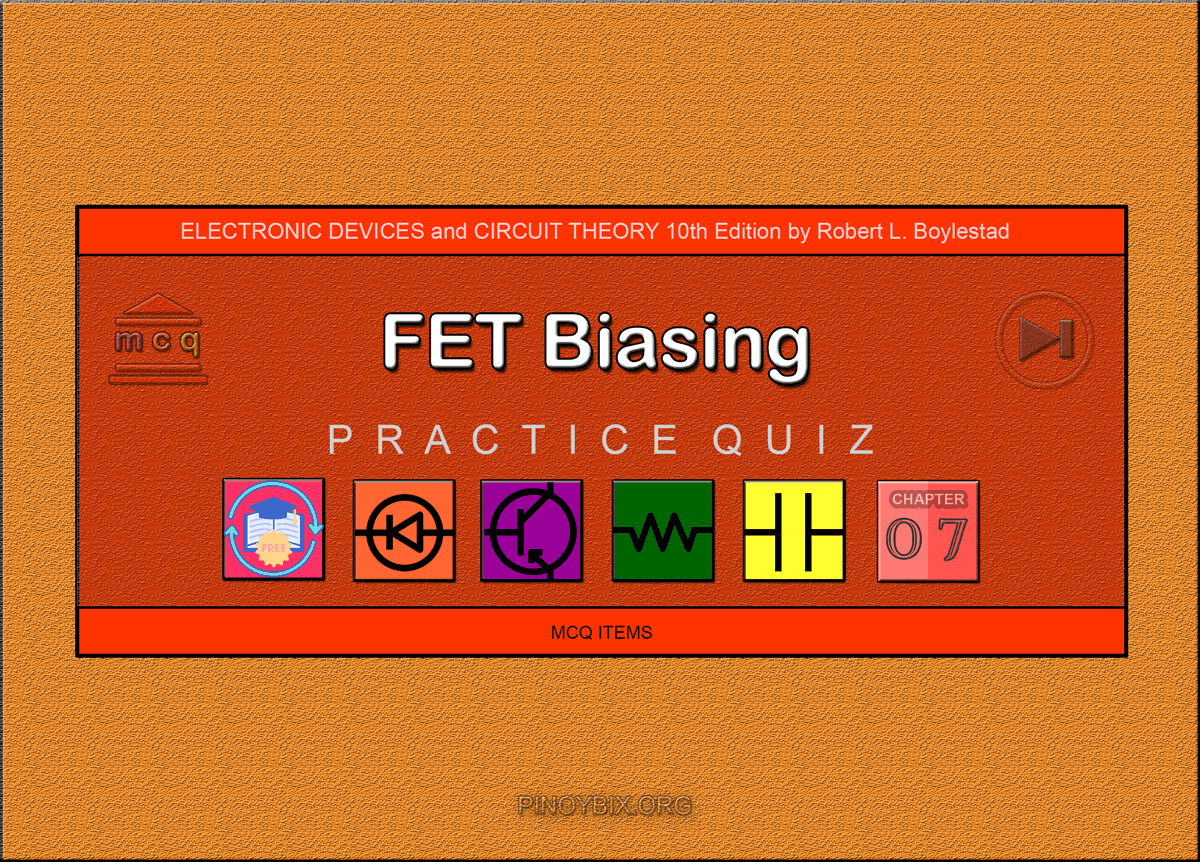This is the Multiple Choice Questions in DC Biasing – FETs from the book Electronic Devices and Circuit Theory 10th Edition by Robert L. Boylestad. If you are looking for a reviewer in Electronics Engineering this will definitely help. I can assure you that this will be a great help in reviewing the book in preparation for your Board Exam. Make sure to familiarize each and every questions to increase the chance of passing the ECE Board Exam.

### Online Questions and Answers Topic Outline

• MCQ in DC Biasing – Field Effect Transistor
• MCQ in Fixed-Bias Configuration
• MCQ in Self-Bias Configuration
• MCQ in Voltage-Divider Biasing
• MCQ in Depletion-Type MOSFETs
• MCQ in Enhancement-Type MOSFETs
• MCQ in Summary Table
• MCQ in Combination Networks
• MCQ in Design
• MCQ in P-Channel FETs
• MCQ in Universal JFET Bias Curve

#### Practice Exam Test Questions

Choose the letter of the best answer in each questions.

1. What is the approximate current level in the gate of an FET in dc analysis?

A) 0 A

B) 0.7 mA

C) 0.3 mA

D) Undefined

Solution:

2. Which of the following current equations is true?

A) IG = ID

B) IG = IS

C) ID = IS

D) IG = ID = IS

Solution:

3. For the FET, the relationship between the input and output quantities is _______ due to the _______ term in Shockley’s equation.

A) nonlinear, cubed

B) linear, proportional

C) nonlinear, squared

Solution:

4. The input controlling variable for a(n) _______ is a current level and a voltage level for a(n) _______.

A) BJT, FET

B) FET, BJT

C) FET, FET

D) BJT, BJT

Solution:

5. Calculate the value of VDS.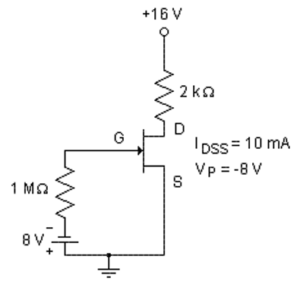A) 0 V

B) 8 V

C) 4.75 V

D) 16 V

Solution:

6. The self-bias configuration eliminates the need for two dc supplies.

A) True

B) False

Solution:

7. Which of the following is (are) true of a self-bias configuration compared to a fixed-bias configuration?

A) One of the dc supplies is eliminated.

B) A resistor RS is added.

C) VGS is a function of the output current ID.

D) All of the above

Solution:

8. Which of the following represents the voltage level of VGS in a self-bias configuration?

A) VG

B) VGS(off)

C) VS

D) VP

Solution:

9. What is the new value of RD when there is 7 V across VDS?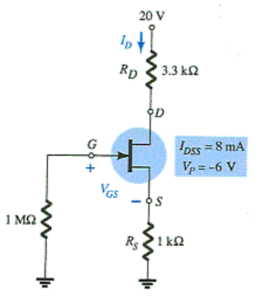A) 3 kΩ

B) 3.3 kΩ

C) 4 kΩ

D) 5 kΩ

Solution:

10. Which of the following is a false statement regarding the dc load line when comparing self-bias and voltage-divider configurations?

A) Both are linear lines.

B) Both cross the origin.

C) Both intersect the transfer characteristics.

D) Both are obtained by writing Kirchhoff’s voltage law (KVL) at the input side loop.

Solution:

11. For what value of RD is the voltage across VDS zero?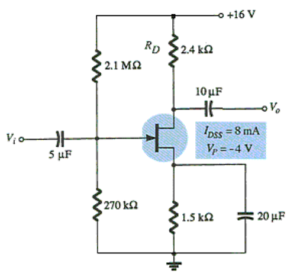A) 2.400 kΩ

B) 5.167 kΩ

C) 6.167 kΩ

D) 6.670 kΩ

Solution:

12. Calculate the value of VDS’.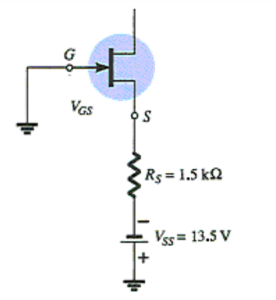A) 0 V

B) 0.35 V

C) 3.8 V

D) 33.5 V

Solution:

13. Which of the following describe(s) the difference(s) between JFETs and depletion-type MOSFETs?

A) VGS can be positive or negative for the depletion-type.

B) ID can exceed IDSS for the depletion-type.

C) The depletion-type can operate in the enhancement mode.

D) All of the above

Solution:

14. At what value of RS does the circuit switch from depletion mode to enhancement mode?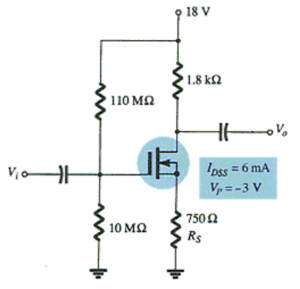A) 250 Ω

B) 500 Ω

C) 10 MΩ

D) None of the above

Solution:

15. For what value of R2 is VGSQ equal to 1 V?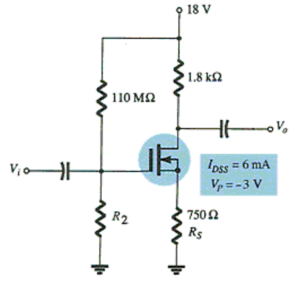A) 10 MΩ`

B) 100 MΩ

C) 110 MΩ

D) 220 MΩ

Solution:

16. Depletion-type MOSFETs do not permit operating points with positive values of VGS and levels of ID that exceed IDSS.

A) True

B) False

Solution:

17. For what value of RS can the depletion-type MOSFETs operate in enhancement mode?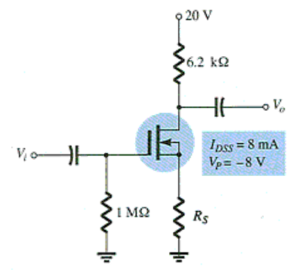A) 2.4 kΩ

B) 5 kΩ

C) 6.2 kΩ

D) None of the above

Solution:

18. Determine the value of VDSQ.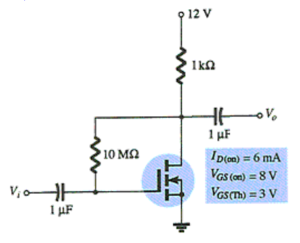A) 3.5 V

B) 4.86 V

C) 7.14 V

D) 10 V

Solution:

19. Calculate the value of VDSQ.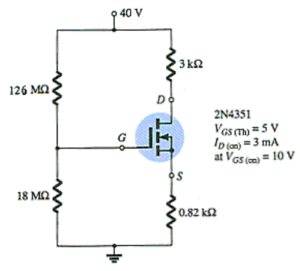A) 0 V

B) 20 V

C) 30 V

D) 40 V

Solution:

20. What are the voltages across RD and RS?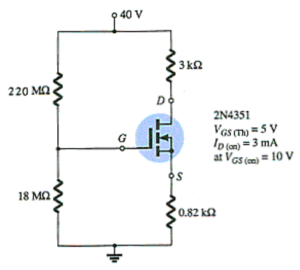A) 0 V, 0 V

B) 5 V, 5 V

C) 10 V, 10 V

D) 20 V, 20 V

Solution:

A) 23.0 V

B) 17.0 V

C) 4.6 V

D) 12.4 V

Solution:

22. Specification sheets typically provide the value of the constant k for enhancement-type MOSFETs.

A) True

B) False

Solution:

A) 0 V

B) 6 V

C) 16 V

D) 11 V

Solution:

A) 0 V

B) 2 V

C) 3 V

D) 5.34 V

Solution:

25. Given the values of VDQ and IDQ for this circuit, determine the required values of RD and RS.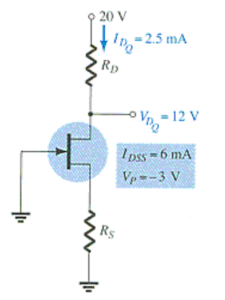A) 2 kΩ, 2 kΩ

B) 1 kΩ, 5.3 kΩ

C) 3.2 kΩ, 400 Ω

D) 2.5 kΩ, 5.3 kΩ

Solution:

26. Calculate the value of RS. Assume VGSQ = −2V.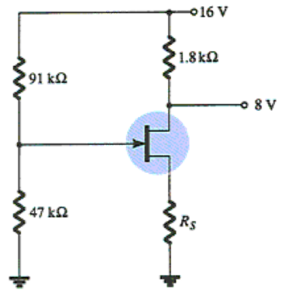A) 0 kΩ

B) 1.68 kΩ

C) 6.81 kΩ`

D) 8.5 kΩ

Solution:

27. Calculate the value of RD’.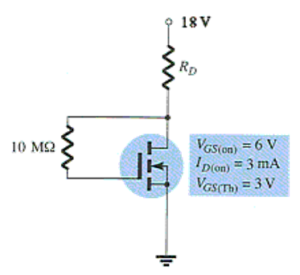A) 2 kΩ

B) 3 kΩ

C) 3.5 kΩ

D) 4.13 kΩ

Solution:

28. In the design of linear amplifiers, it is good design practice to choose operating points that do not crowd the saturation level or cutoff regions.

A) True

B) False

Solution:

29. Seldom are current levels measured since such maneuvers require disturbing the network structure to insert the meter.

A) True

B) False

Solution:

30. Calculate the value of VDS.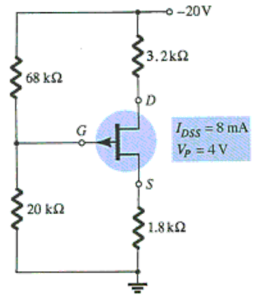A) –3 V

B) 3 V

C) –4 V

D) 4 V

Solution:

31. Determine the quiescent values of ID and VGS.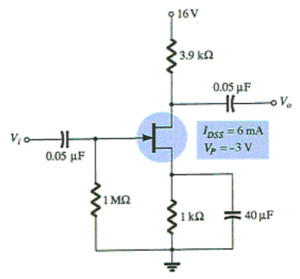A) 1.2 mA, –1.8 V

B) 1.5 mA, –1.5 V

C) 2.0 mA, –1.2 V

D) 3.0 mA, –0.8 V

Solution:

A) 1.0 V

B) 1.50 V

C) 2.56 V

D) 3.58 V

Solution:

33. On the universal JFET bias curve, the vertical scale labeled _______ can, in itself, be used to find the solution to _______ configurations.

A) m, fixed-bias

B) M, fixed-bias

C) M, voltage-bias

D) m, voltage-bias

Solution:

34. Through proper design, a ________ can be introduced that will affect the biasing level of a voltage-controlled JFET resistor.

A) photodiode

B) thermistor

C) laser diode

D) Zener diode

Solution:

35. For the non-inverting amplifier, one of the most important advantages associated with using a JFET for control is the fact that it is _______ rather than _______ control.

A) dc, ac

B) ac, dc

C) any of the above

D) none of the above

Solution:

#### Fill-in-the-blanks Questions

1. For the field-effect transistor, the relationship between the input and the output quantities is _______.

A) linear

B) nonlinear

C) 3rd degree

D) None of the above

Solution:

2. The input controlling variable for an FET transistor is a _______ level.

A) resistor

B) current

C) voltage

D) All of the above

Solution:

3. The controlled variable on the output side of an FET transistor is a _______ level.

A) current

B) voltage

C) resistor

D) None of the above

Solution:

4. For _______, Shockley’s equation is applied to relate the input and the output quantities.

A) JFETs

B) depletion-type MOSFETs

C) enhancement-type MOSFETs

D) JFETs and depletion-type MOSFETs

Solution:

5. The coupling capacitors are _______ for the dc analysis and _____________ for the ac analysis.

A) open-circuit, low impedance

B) short-circuit, low impedance

C) open-circuit, high impedance

D) None of the above

Solution:

6. In a fixed-bias configuration, the voltage level of VGS is equal to _______.

A) VS

B) VG

C) VGS(off)

D) VP

Solution:

7. The ratio of current ID to IDSS is equal to _______ for a fixed-bias configuration.

A) 0

B) 0.25

C) 0.5

D) 1

Solution:

8. When plotting the transfer characteristics, choosing VGS = 0.5VP will result in a drain current level of _______ IDSS.

A) 0

B) 0.25

C) 0.5

D) 1

Solution:

9. The dc load line is drawn using the equation obtained by applying Kirchhoff’s voltage law (KVL) at _______ side loop(s) of the circuit.

A) the output

B) the input

C) both the input and output

D) None of the above

Solution:

10. The slope of the dc load line in a self-bias configuration is controlled by _______.

A) VDD

B) RD

C) RG

D) RS

Solution:

11. ______ levels of RS result in _______ quiescent values of ID and _______ negative values of VGS.

A) Increased, lower, less

B) Increased, higher, less

C) Increased, higher, more

D) Increased, less, lower

Solution:

12. The slope of the dc load line in a voltage-divider is controlled by _______.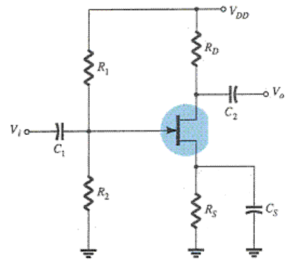A) R1

B) R2

C) RS

D) All of the above

Solution:

13. In a depletion-type MOSFET, the transfer characteristic rises ________ as VGS becomes more positive.

A) less rapidly

B) more rapidly

C) the same

D) None of the above

Solution:

14. In ________ configuration(s) a depletion-type MOSFET can operate in enhancement mode.

A) self-bias

B) fixed-bias with no VGG

C) voltage-divider

D) None of the above

Solution:

15. In an enhancement-type MOSFET, the drain current is zero for levels of VGS less than the _______ level.

A) VGS(Th)

B) VGS(off)

C) VP

D) VDD

Solution:

16. Specification sheets typically provide ________ for enhancement-type MOSFETs.

A) the threshold voltage VGS(Th)

B) a level of drain current ID(on)

C) an ID(on)

D) All of the above

Solution:

17. In a feedback-bias configuration, the slope of the dc load line is controlled by ________.

A) RG

B) RD

C) VDG

D) None of the above

Solution:

18. For R2 smaller than ________ kΩ the voltage VD is equal to VDD = 16 V.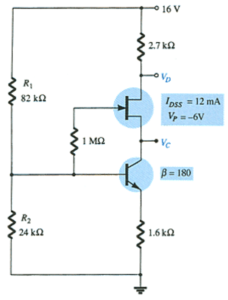A) 3.75

B) 5

C) 12.0

D) 24

Solution:

19. ________ must be considered in the total design process.

A) Dc conditions

B) Level of amplification

C) Signal strength

D) All of the above

Solution:

20. In a JFET, the level of ________ is limited to values between 0 V and –VP.

A) VSQ

B) VDGQ

C) VDSQ

D) VGSQ

Solution:

21. The level of VDS is typically between _____ % and _____ % of VDD.

A) 0, 100

B) 10, 90

C) 25, 75

D) None of the above

Solution:

22. In a universal JFET bias curve, the vertical scale labeled m is used to find the solution to the ________ configuration.

A) fixed-bias

B) self-bias

C) voltage-divider

D) None of the above

Solution:

23. In a universal JFET bias curve, the vertical scale labeled M is used for finding the solution to the ________ configuration.

A) fixed-bias

B) self-bias

C) voltage-divider

D) None of the above

Solution:

24. In a universal JFET bias curve, the horizontal axis is _______.

A) VDS

B) ID / IDSS

C) the normalized level VGS/|VP|

D) VGS

Solution:

25. In p-channel FETs, the level of VGS is _______ while the level of VDS is _______.

A) negative, negative

B) positive, positive

C) negative, positive

D) positive, negative

Solution:

### Complete List of Chapter Quiz in Electronic Devices and Circuit Theory

Help Me Makes a Difference!

 P inoyBIX educates thousands of reviewers/students a day in preparation for their board examinations. Also provides professionals with materials for their lectures and practice exams. Help me go forward with the same spirit. “Will you make a small \$5 gift today?” Option 1 : \$1 USD Option 2 : \$3 USD Option 3 : \$5 USD Option 4 : \$10 USD Option 5 : \$25 USD Option 6 : \$50 USD Option 7 : \$100 USD Option 8 : Other Amount© 2014 PinoyBIX Engineering. © 2019 All Rights Reserved | How to Donate? |#### GEAS Solution

Dynamics problem Economics problem Physics problem Statics problem Strength problem Thermodynamics problem

#### Questions and Answers in GEAS

Engineering Economics Engineering Laws and Ethics Engineering Management Engineering Materials Engineering Mechanics General Chemistry Physics Strength of Materials Thermodynamics
Consider Simple Act of Caring!: LIKE MY FB PAGE

Our app is now available on Google Play, Pinoybix Elex